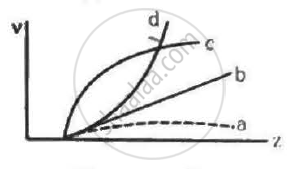Department of Pre-University Education, KarnatakaPUC Karnataka Science Class 12
Advertisement Remove all ads

# Frequencies of Kα X-rays of Different Materials Are Measured. Which One of the Graphs in the Figure May Represent the Relation Between the Frequency V and the Atomic Number Z ? - Physics

Graph

Frequencies of Kα X-rays of different materials are measured. Which one of the graphs in the figure may represent the relation between the frequency v and the atomic number ?Advertisement Remove all ads

#### Solution

Using Moseley's Law,

sqrt(v) = a(Z - b),

where v = frequency of Kα X-ray
Z = atomic number

therefore  v = a^2(Z - b)^2

⇒ (Z - b)^2 = v/a^2

This is  the equation of a parabola with some intercept on the axis, representing atomic number (Z). Hence, curve represent this relation correctly.

Is there an error in this question or solution?
Advertisement Remove all ads

#### APPEARS IN

HC Verma Class 11, Class 12 Concepts of Physics Vol. 2
Chapter 22 X-rays
MCQ | Q 7 | Page 394
Advertisement Remove all ads

#### Video TutorialsVIEW ALL 

Advertisement Remove all ads
Share
Notifications

View all notifications

Forgot password?